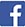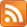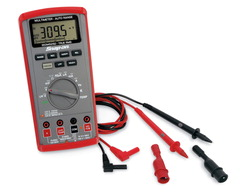WELCOME!Cruzer's RV TechMag is the ultimate source for technical articles, travel destinations, road tests and reviews as well as the latest and greatest RV products and accessories and editorial commentary related to the RV lifestyle. You may choose from the following selections to narrow down your area of interest.

 All Articles Reviews Tech Travel Misc Highway Tales

# RV Electrical Tutorial## Chapter 2 - Amps, Volts, and Watts

In the previous chapter we talked about how electricity functions in a general sense. We also touched briefly on amps, volts, and the differences between AC and DC current. In this chapter we'll talk about voltage, amperage, wattage, and how various electrical loads all affect this as well as how to measure electricity.

Voltage

As we mentioned in last chapter, voltage is the force or pressure exerted upon the electrons to make them flow. The higher the voltage, the easier it is too move them and draw energy from them. Low voltage doesn't have much force or "push", which is why you can touch a small 9 volt battery with your fingers and not feel it. If you touch it to your tongue you will be able to feel it tingle as the current flows through the saliva on your tongue because the saliva conducts electricity. If you touch a hot 12 volt automotive wire you won't feel it either but if you touch a 120 volt electrical wire you'll get a shock which could even be fatal. The higher voltage helps to push the current through where a lower voltage just isn't enough to overcome the resistance in the conductor. 12 volts flows fine through a copper wire because it's good at conducting electricity but the skin on your hand isn't that great of a conductor so you don't feel it if you touch the terminals of a 12 volt battery.

Wire is used to conduct electricity to where it is supposed to go. In order to contain that electricity inside the wire it needs to be insulated. The insulation needs to be capable of preventing any short circuits between the inner conductor and anything that it pass nearby. As the voltage increases, so does the quality of the insulation. Automotive wire is commonly called low voltage wire because it's good for handling 12 to 24 volts but it won't contain a higher 120 volt current. High voltage wire, as used in residential construction, is available in two version. The full version is rated at 600 volts while the junior version is rated at 300 volts. Either will work for a 120 to 240 volt AC circuit but the 600 volt wire has a heavier and better quality insulation. High voltage wire can be used in a low voltage situation but low voltage wire can never be used in a high voltage situation.Voltage is measured by a voltmeter. This can be a dedicated unit or a feature within a multi-meter. Voltmeters that are mounted in an RV are a single function display while the vast majority of portable test equipment is found in the form of a multi-meter, which combines voltage with other measurements, such as amperage, resistance, etc. This popular item is really something that every RVer should have in their toolbox because it can be useful in many different situations.

Amperage

We also talked about amperage in the last chapter. Whereas voltage represents how hard the "push" is upon the flow of electrons, amperage represents the actual volume, or "quantity", of electrons in that flow. This is measured by an ammeter that can either be mounted into an RV control panel or it may be part of a portable multi-meter. Amperage is current flow and just like water flow, it needs an adequate sized conductor or "pipe" to flow through. The larger the flow, the larger the pipe diameter needs to be. Wire is measured in Wire Gauge sizes, sometimes referred to as American Wire Gauge (AWG) when sizes are given. The wire diameter gets smaller as the number gets larger, so a #12 wire is larger than a #16. When you get to large diameter cables, such as used for batteries or welders, you'll eventually run out of numbers at zero. At that point you start doubling digits so a #00 cable is larger than a #0 cable but smaller than a #0000 cable. After that it gets more complex but we don't need to go there because RVs don't have that large of wiring and #0000 is as large as you'll ever see.

Wire gauge size has nothing to do with voltage. All it's concerned with is the amperage, or current flow. But, as we previously mentioned, the insulation value is voltage sensitive. This means that a #0 battery cable designed for 12 volts will not have sufficient insulation to contain a 120 or 240 volt current so it is not to be used for high voltage applications. Both a high voltage and low voltage cable of the same diameter will be able to pass the same amperage but not the same voltage.

Wattage:

Okay, we now know what voltage is and what amperage is. Now we need to see what the relationship is between them and why we just don't have one voltage to run everything so as to keep it simple. That's where wattage comes in. Wattage is the result of multiplying amps times volts. In other words a light bulb that consumes 5 amps at 12 volts is a 60 watt light bulb, because 5 times 12 equals 60. Wattage is considered True Power and that's the part that is important because it's wattage that performs the work, not amperage or voltage. Let's look at a high voltage application as an example.

We'll assume that there is a device, such as an electric air compressor, that needs 3,600 watts of power to operate. If we want to plug this into a 120 volt wall outlet we will first need to find out how big of a circuit we need to run it. We know that amps times volts equal watts therefore watts divided by volts will give us amps. By dividing 3,600 watts by 120 volts we see that we will need a 30 amp circuit to run this unit. The typical duplex receptacle uses a #12 wire with a 20 amp circuit breaker so that's not going to happen. We either need to supply it with a larger #10 wire outlet and 30 amp breaker or change the parameters of our equation. We can do this by changing the voltage. By rewiring the air compressor so that it runs on 240 volts we will reduce the amperage running through the compressor. Using our formula once again we can divide 3,600 watts by 240 volts and we now see that we only need a 15 amp circuit, because 3,600 divided by 240 equals 15. By doubling the voltage we've effectively cut the amperage in half. When we reduce the amperage we also can reduce the wire gauge size down from a #10 wire to a #14 wire because the wire gauge is not interested in voltage, only amperage. That's the reason why utility companies move huge amounts of current through 40,000 volt wires and then step it down to a more useable voltage via transformers and sub-stations. If they ran everything through at 120 volts the wires would probably be the size of a freight train. Yet high voltage requires serious insulation, which is why those huge glass insulators are used on utility poles. Those just aren't practical for wiring inside an RV so lower voltage insulated wires are used instead.

Resistance:

The most popular formula in basic electricity is called Ohm's Law, which is named after the German physicist Georg Ohm. It simply states that E = I * R, or Voltage (E) equals Amperage (I) times Resistance (R). Resistance is just how hard it is to pass electricity through. It's measured via an Ohmmeter or multi-meter and expressed in Ohms. A 2x4 hunk of wood has very high resistance so wood does not make a good conductor of electricity. Silver is the best but for obvious reasons (cost) it doesn't get used very often. Copper is the most popular conductor and the majority of the wiring out there will be copper because it has low resistance. If we add a tungsten or nichrome wire to our circuit, it will have higher resistance. As current passes through a tungsten wire that resistance will cause it that wire to glow if it is kept in a vaccum or surrounded by an inert gas therefore it will make good light bulb filament. Nichrome is commonly used as a heating element, such as in a toaster. The greater the resistance, the lesser the light or heat output. For example, a 60 watt light bulb running on a 120 volt circuit consumes .5 amps (60 watts / 120 volts = .5 amps). Using Ohm's law we see that the resistance equals voltage divided by current (amperage) so our light bulb has 240 ohms of resistance (120 volts / .5 amps = 240 ohms). If we look at a brighter 150 watt bulb we see that it needs 1.25 amps to run (150 watts / 120 volts = 1.25 amps). Using Ohm's law we get 96 ohms as the resistance (120 volts / 1.25 amps = 96 ohms). This shows us that we get more light (wattage) out of a higher flowing filament with less resistance than with a high resistance lower flowing filament. This formula can also be used for testing other components. You can use these formulas to determine if an electric heating element, such as a hot water heater, is functioning as it should. Simply find the wattage of the water heater, divide by the voltage to get its amperage. Then take the voltage, divide it by the amperage to get what the resistance of the water heater element should be. Then test the element with an ohmmeter to see what it reads. It should be reasonable close to your calculations. If not, your element isn't heating as it should and probably needs to be replaced.

Resistance also affects voltage. The AC components in your RV are designed to operate on 120 volts. There is some tolerance, plus or minus 10%, but if the voltage goes higher or lower than that you can damage your equipment. If you run too much current through too small of a wire or cord, the voltage drops. Using Ohm's law we can see why. Let's assume we have a 100' long #12 gauge cord which has a resistance of 6 ohms. This is capable of passing 20 amps of current at 120 volts (120 volts / 6 ohms = 20 amps). When we try to pass 30 amps of power through this cord, which is only rated for 20 amps, we get a voltage drop because the extra current cannot overcome the resistance. We would need a larger #10 gauge cord with 4 ohms of resistance in order to pass 30 amps of power. If we try to pass 30 amps through the smaller cord the voltage will drop and the amperage will rise. This will cause the cord to heat up, possibly starting a fire. For this reason circuit breakers must always be sized for the proper size wire gauge and never replace with a larger breaker than that wire can safely handle.

In the next section we'll talk about the differences between a 30 amp service and 50 amp service.

## Index

 Return to Beginning Go to Last Chapter - Introduction to Electricity Go to Next Chapter - 30 Amp versus 50 Amp